You are here: Home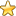Esoteric Wisdom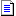# The Simplexes

Of the Regular Polytopes The simplest shape in any dimension is called a simplex. The diagrams below show that the simplexes, the partitions of n and the permutations of yin and yang are intimately related. It is my belief that there is also a link with the Elemental Concepts.Beyond dimensional space is Oneness.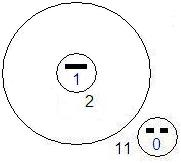The simplest regular shape in 0 dimensional space is the point.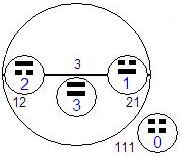The simplest regular shape in 1 dimensional space is the line.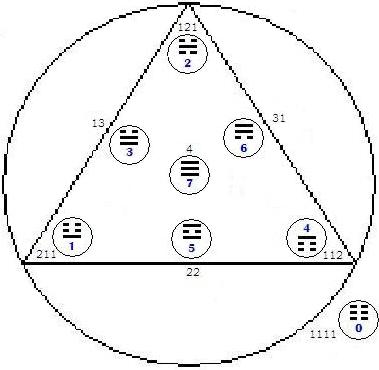The simplest regular shape in 2 dimensional space is the equilateral triangle.It adds to 14 in every direction through the center.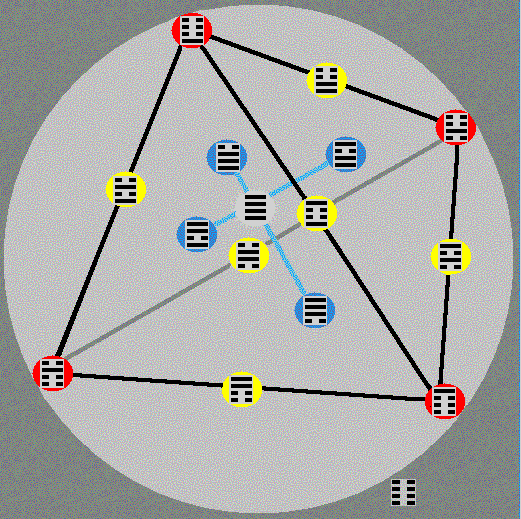The simplest regular shape in 3 dimensional space is the tetrahedon.

The broken and single horizontal lines in the above diagrams symbolize yin and yang respectively. They also represent base 2 numeration thus: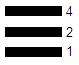The unbroken yang lines represent 1 and the broken lines represent 0. so the trigram above being composed of 3 solid lines has a value of 1+2+4=7. In common binary notation it would be written as 111.

You are here: HomeEsoteric WisdomSearch on Amazon:
simplexes or...?